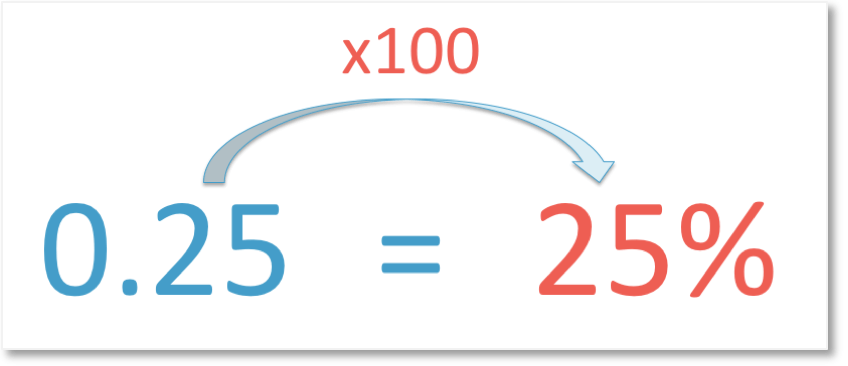# How to Convert Centimetres to Millimetres (cm to mm)

How to Convert Centimetres to Millimetres (cm to mm)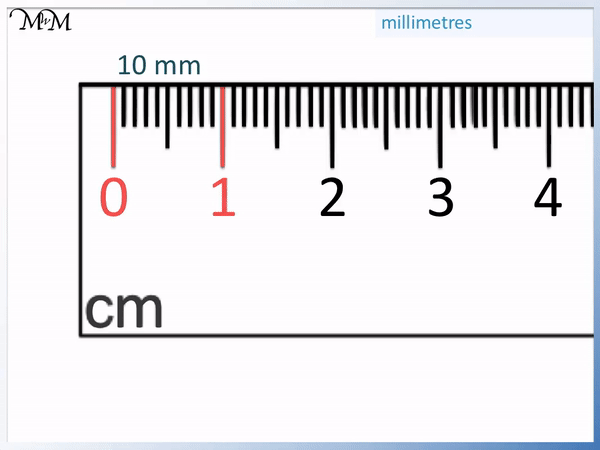• Each number on a ruler represents one centimetre.
• Centimetres are written as ‘cm’ for short.
• The distance between the smallest lines on a ruler is 1 millimetre.
• Millimetres are written as ‘mm’ for short.
• There are 10 millimetres in each centimetre.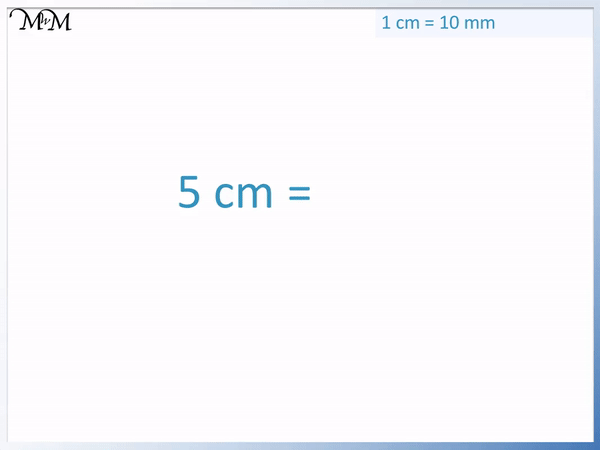• Each centimetre is worth 10 millimetres.
• To convert from cm to mm, multiply the value in centimetres by 10.
• 5 multiplied by 10 is 50.
• 5 cm is the same length as 50 mm.
To convert cm to mm, multiply by ten.• To convert centimetres to millimetres, multiply by 10.
• 2.8 multiplied by 10 is 28.
• 2.8 cm is the same length as 28 mm.
• 28 mm is shown on the ruler above.
• This position is 28 millimetres away from zero.
• 28 mm is eight millimetres away from the 2 cm mark.Supporting Lessons

#### Converting cm to mm with Decimals Video Lesson# How do we Convert cm to mm (Centimetres to Millimetres)?

## How to Convert Centimetres to Millimetres

To convert centimetres to millimetres, multiply by 10. Every centimetre is worth 10 millimetres. For example, 5 cm = 50 mm because 5 × 10 = 50.

5 cm is the same as 50 mm.

5 cm = 50 mmTo multiply a whole number by 10, we can simply write a 0 on the end. 5 cm becomes 50 mm.

This trick to multiply by 10 will only work for whole numbers and will not work for decimal values.

Here is an example of converting centimetres to millimetres with a decimal number.

We are asked to convert 1.2 centimetres into millimetres. To convert cm to mm, we multiply the value by ten.

1.2 x 10 = 12

So, 1.2 cm is the same as 12 mm.

1.2 cm = 12 mm

We can see where 1.2 cm and 12 mm lie on the ruler in the clip below.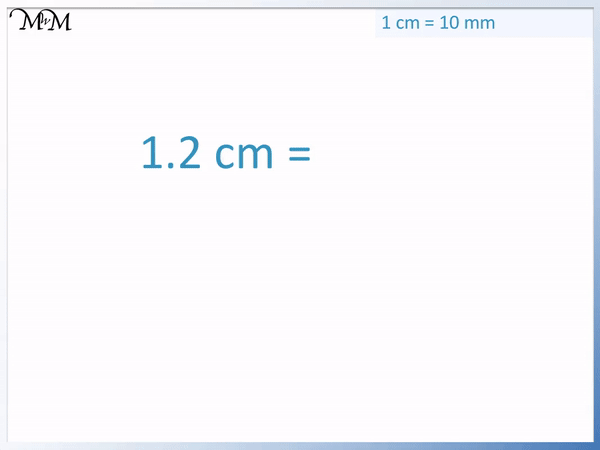Notice that if we simply ‘added a zero’ to the end of the 1.2 decimal value, this would not have multiplied it by ten. We would have calculated an incorrect answer.

This is because 1.2 is the same value as 1.20.

A trick to help us multiply a number with only one decimal place can be to move the digit after the decimal point in front of the decimal point.

This has the same effect as simply removing the decimal point from our answer.

1.2 cm becomes 12 mm.

If you need further practice of multiplying a decimal number by 10 and wish to understand why this trick works, please watch our lesson on Multiplying by 10, where we explore this topic further.

In the following example, we have a decimal number. We are asked to convert 2.8 centimetres into millimetres. To convert cm to mm, we multiply by ten.

2.8 x 10 = 28

So, 2.8 cm is the same as 28 mm.

2.8 cm = 28 mm

We can see where 2.8 cm and 28 mm lie on the ruler in the clip below.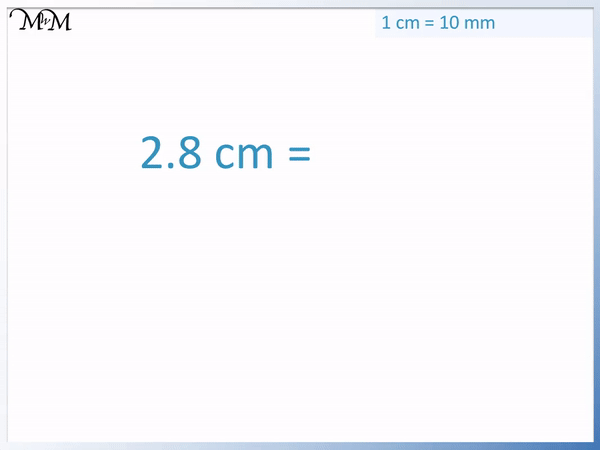Again notice that ‘adding a zero’ will not work for multiplying a decimal number by 10.

Instead, you could use the trick for numbers that contain only one decimal place and remove the decimal point.

28 mm is shown on the ruler above as 28 individual millimetre marks away from the zero mark or simply 8 mm away from the 2 cm mark.

## Centimetres to Millimetres on a Ruler

Each number written on a ruler is in centimetres. Millimetres are the smallest lines shown on a ruler in between each centimetre. There are ten millimetre lines between each centimetre.

Centimetres are written as ‘cm’ for short.

Millimetres are written as ‘mm’ for short.

Write a space between the number and the ‘mm’ or ‘cm’.

In the following example, we are asked to convert 8 cm into millimetres. We will convert cm to mm using a ruler.

To convert a length in centimetres into a length in millimetres, multiply the value in centimetres by ten.

To convert cm to mm, we multiply by ten.

8 x 10 = 80

So, 8 cm is the same as 80 mm.

8 cm = 80 mmIn the following example, we are asked to convert 11 cm into millimetres. To convert cm to mm, we multiply by ten.

11 x 10 = 110

So, 11 cm is the same as 110 mm.

11 cm = 110 mm## Teaching Converting cm to mm

When teaching converting centimetres to millimetres, it is helpful to use a large ruler to show the mm and cm increments. Using a large ruler or an interactive ruler online can be an easier way to show the comparison because mm on real rulers can be very small.

Below, we have a 15 cm ruler. We use it to measure a length in centimetres.

The distance between each number that is written on the ruler is one centimetre (1 cm).The smaller divisions between each number are millimetres (mm).

The millimetre markings are the smallest lines on the ruler.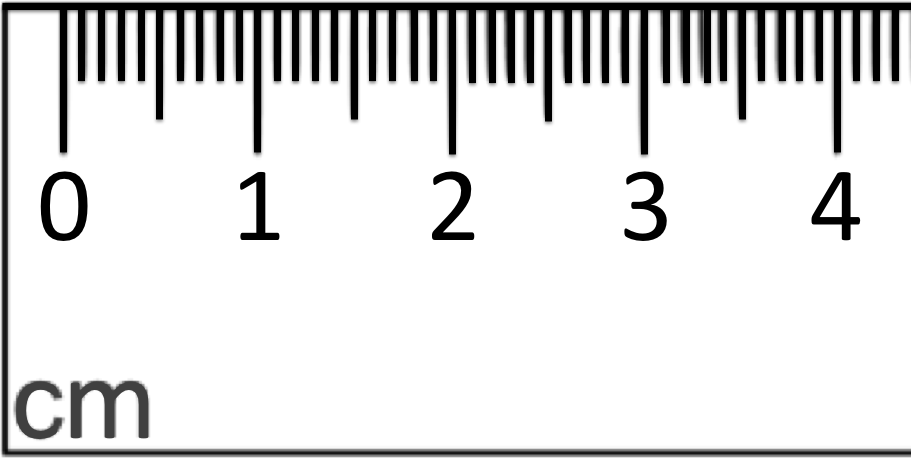The distance between 0 and the first mark is one millimetre (1 mm).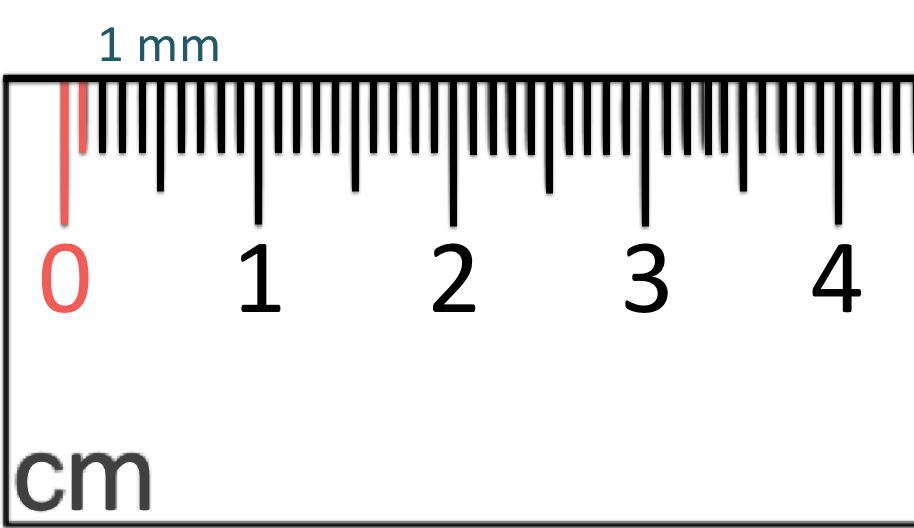The distance between 0 and the second line is two millimetres (2 mm).

The distance between 0 and the third line is three millimetres (3 mm).

This continues as we count along the millimetre lines of the ruler as can be seen in the image below.Ten millimetres is at the same mark as the one centimetre mark.

So, we can see that 1 cm is the same length as 10 mm.

To convert from 1 cm to 10 mm, we multiply by ten.

There are 10 millimetres in one centimetre.

1 x 10 = 10

So, to convert centimetres to millimetres (to convert cm to mm), multiply the value in centimetres by ten.Now try our lesson on Converting Decimals to Percentages where we learn how to convert a decimal number to a percentage.